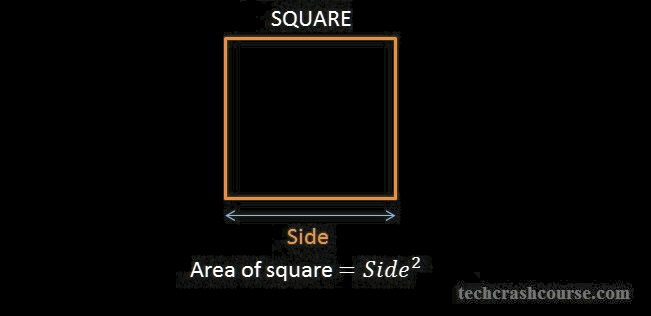# Square root

## Introduction

When you try to get the area of a square noteA shape where all sides are equal and know the length of a side noteOtherwise known as its root you multiply the length of one side by itself or square it.What if you don’t know the length of a side and instead only know the area of a square and want to get the length of a side? You would have to do something to the area that transforms it in the opposite direction that squaring the length of a side does. This is just like how placing 2 apples to a pile transforms a pile of apples by increasing the amount of apples and eating 2 apples transforms the pile back to the way it was. So in other words adding is to subtracting as squaring is to…. what?

Well this kind of transformation is called a Square Root.

Parents:

• Mathematics

Mathematics is the study of numbers and other ideal objects that can be described by axioms.